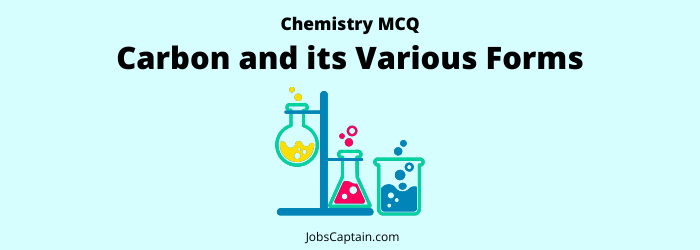# MCQ on Carbon and its Various Forms Quiz: ChemistryQuestion 1. Consider the following statements and select the correct answer from given codes below.

1. Water becomes harder due to the presence of Calcium Sulphate and it is not usable.
2. Diamond is harder than copper and iron.
3. Oxygen is the main component of air.
4. Nitrogen is used in the manufacture of vegetable ghee.

Code

(A) 1 and 2

(B) 3 and 4

(C) 1 and 3

(D) 2 and 4

(A) 1 and 2

Question 2. Graphite was in news recently. What is its importance?

1. It is a two-dimensional material and has good electrical conductivity.
2. It is one of the thinnest but strongest materials tested so far.
3. It is entirely made of silicon and has high optical transparency.
4. It can be used as conducting electrodes required for touch screens, LCD’s and organic LED’s.

Which of the statement(s) given above is/are correct?

(A) 1, 2, 3 and 4

(B) 1, 2 and 4

(C) 3 and 4

(D) 1 and 2

(B) 1, 2 and 4

Question 3. Consider the following statements. Glass can be etched or scratched by:

1. Diamond
2. Hydrofluoric Acid
3. Aquarregia
4. Conc. Sulphuric Acid

Which of these statements are correct?

(A) 2 and 4

(B) 1 and 2

(C) 2 and 3

(D) 1 and 4

(B) 1 and 2

Question 4. Water gas is _________.

(A) CO2 + H2O

(B) CO2 + H2

(C) CO + H2O

(D) CO + H2

(D) CO + H2

Question 5. Which one of the following nonmetals is not a poor conductor of electricity?

(A) Phosphorus

(B) Bromine

(C) Selenium

(D) Sulphur

(C) Selenium

Question 6. Chemically Dry Ice is ___________.

(A) Solid carbon dioxide

(B) Mixture of ice and common salt

(C) Ice made from distilled water

(D) Solid sulphur

(A) Solid carbon dioxide

Question 7. Which lubricant is used for heavy machines?

(A) Silicon oil

(B) Graphite

(C) Phosphorus

(D) Bauxite

(B) Graphite

Question 8. Which one of the following materials is strongest?

(A) Graphene

(B) Steel

(C) Brass

(D) German Silver

(A) Graphene

Question 9. For the ceiling of diamonds, the unit of weight is carat. One carat is equal to _________.

(A) 400 mg

(B) 300 mg

(C) 200 mg

(D) 100 mg

(C) 200 mg

Question 10. Which of the following is not in the form of crystal?

(A) Graphite

(B) Sulphur

(C) Quartz

(D) Diamond

(B) Sulphur

Question 11. The highest amount of carbon is in __________.

(A) Alloy Steel

(B) Steel

(C) Wrought Iron

(D) Pig Iron

(D) Pig Iron

Question 12. Which one of the following types of coal contains a higher percentage of Carbon than the rest type of _________.

(A) Anthracite

(B) Peat

(C) Lignite

(D) Bituminous coal

(A) Anthracite

Question 13. Which of these consists carbon?

(A) Iron

(B) Silver

(C) Tin

(D) Lignite

(D) Lignite

Question 14. Which of the following do not contain carbon?

(A) Sand

(B) Coal

(C) Graphite

(D) Diamond

(A) Sand

Question 15. Buckminster Fullerene is ___________.

(A) A form of carbon compound of clusters of 60 carbon atoms bond together in polyhedral structure composed of pentagons or hexagons

(B) A polymer of fluorine

(C) An isotope of carbon heavier than C14

(D) None of the above

(A) A form of carbon compound of clusters of 60 carbon atoms bond together in polyhedral structure composed of pentagons or hexagons

Question 16. Third allotrope of Carbon was discovered by three scientists, who were awarded the Nobel Prize for Chemistry. Find out who was not on that team.

(A) Faimen

(B) R.E. Smalley

(C) R.F. Curl

(D) H.W. Kroto

(A) Faimen

Question 17. Pencil lead is ______________.

(A) Coal

(B) Lamp black

(C) Charcoal

(D) Graphite

(D) Graphite

Question 18. Which of the following do not consist carbon?

(A) None of these

(B) Coal

(C) Graphite

(D) Diamond

(A) None of these

Question 19. Which one of the following elements forms the maximum number of compounds?

(A) Oxygen

(B) Nitrogen

(C) Carbon

(D) Hydrogen

(C) Carbon

Question 20. Which of these is not an additional form of Carbon?

(A) Fullerenes

(B) Oxocarbon

(C) Graphite

(D) Diamond

(B) Oxocarbon

Question 21.  Which of the following is made up of Carbon only?

(A) Spider silk

(B) Graphene

(C) Lexan

(D) Kevlar